# Introduction

The newest version of ggplot2 3.2.0 gave us the ability to change the glyph in the legend like so

``````library(ggplot2)

ggplot(economics_long, aes(date, value01, colour = variable)) +
geom_line(key_glyph = "timeseries")``````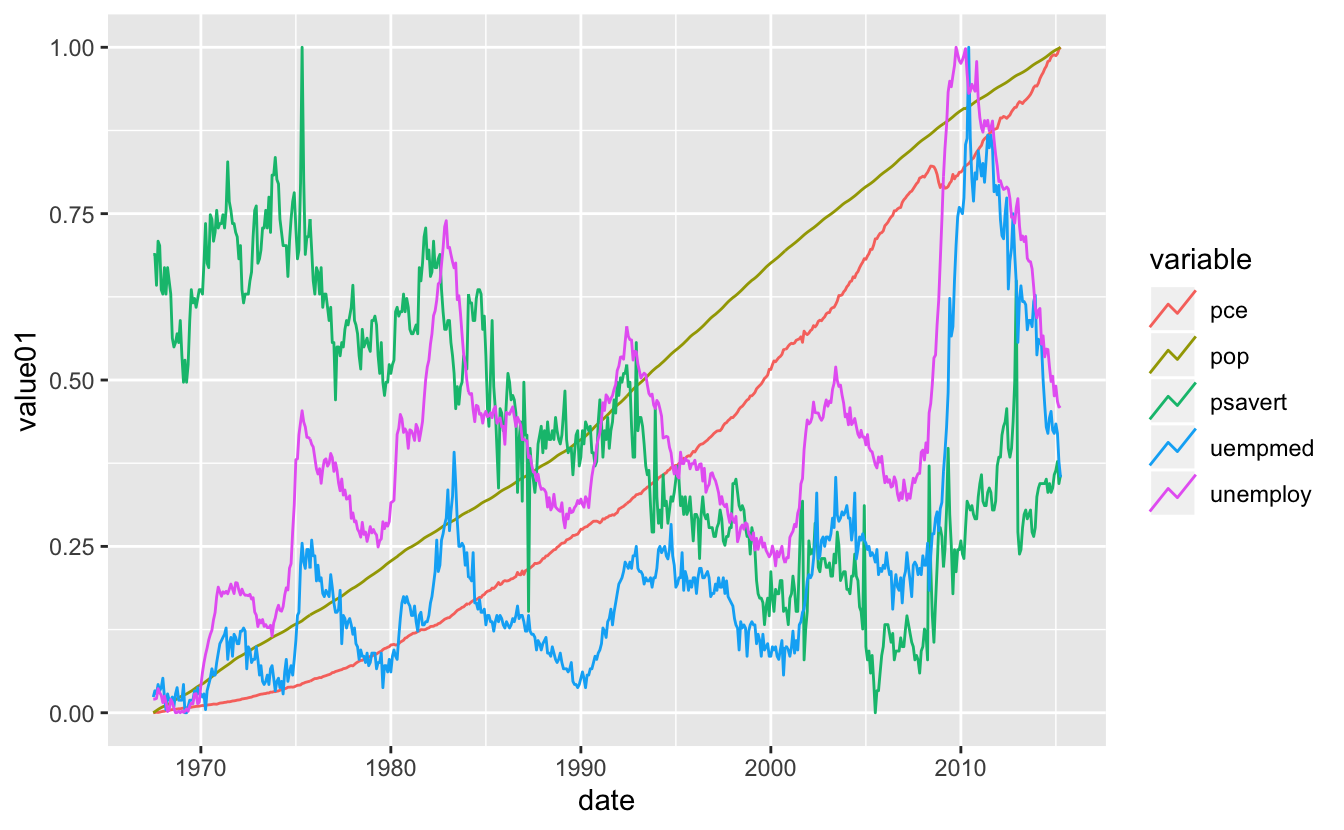And they can likewise be specified with the `draw_key_*` functions as well

``````ggplot(economics_long, aes(date, value01, colour = variable)) +
geom_line(key_glyph = draw_key_timeseries)``````# Showcase

The following is all the available `draw_key_*` functions in ggplot2. Notice that the dark gray color in dotplot and polygon is a result of a unspecified `fill` aesthetic. Code to generate these figures can be found at the end of this post.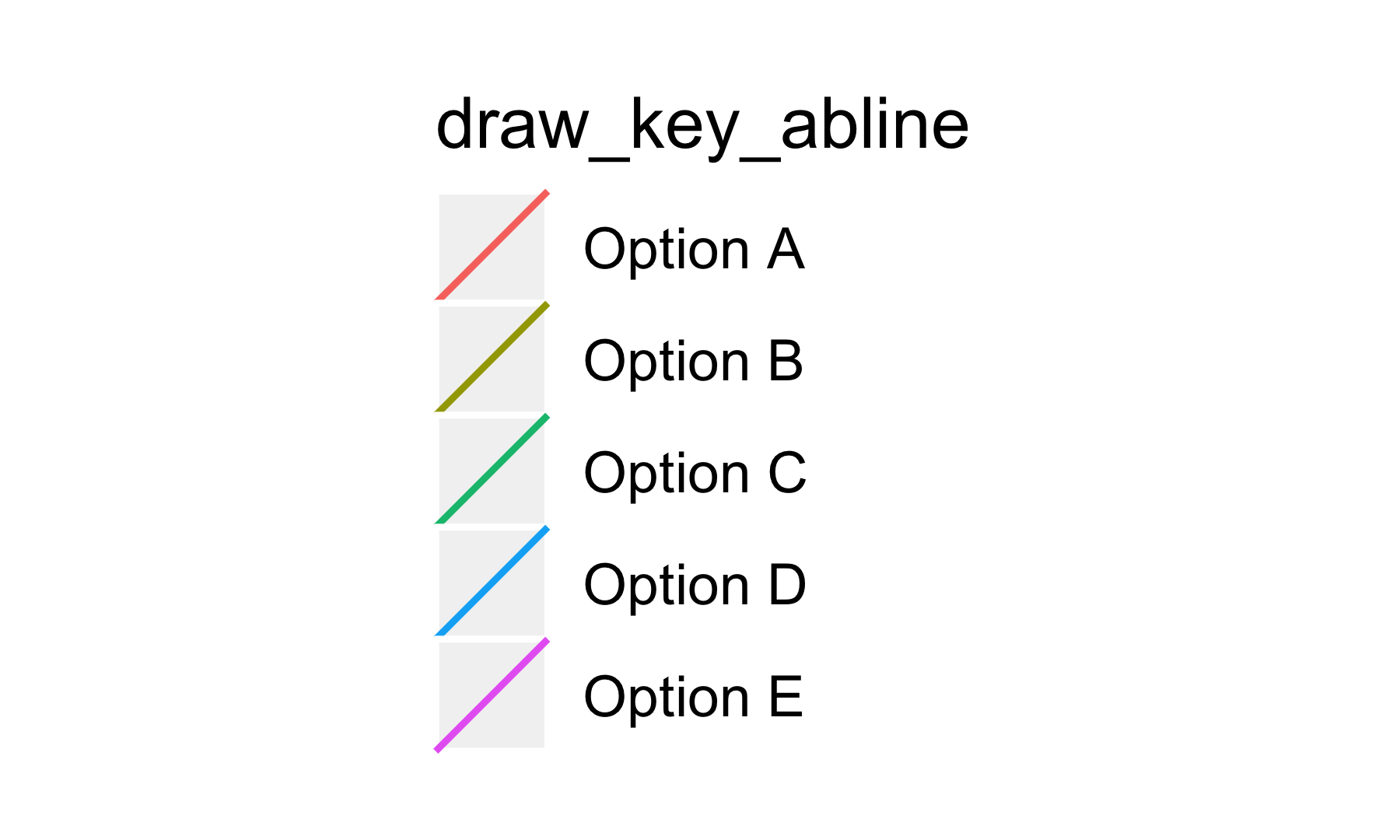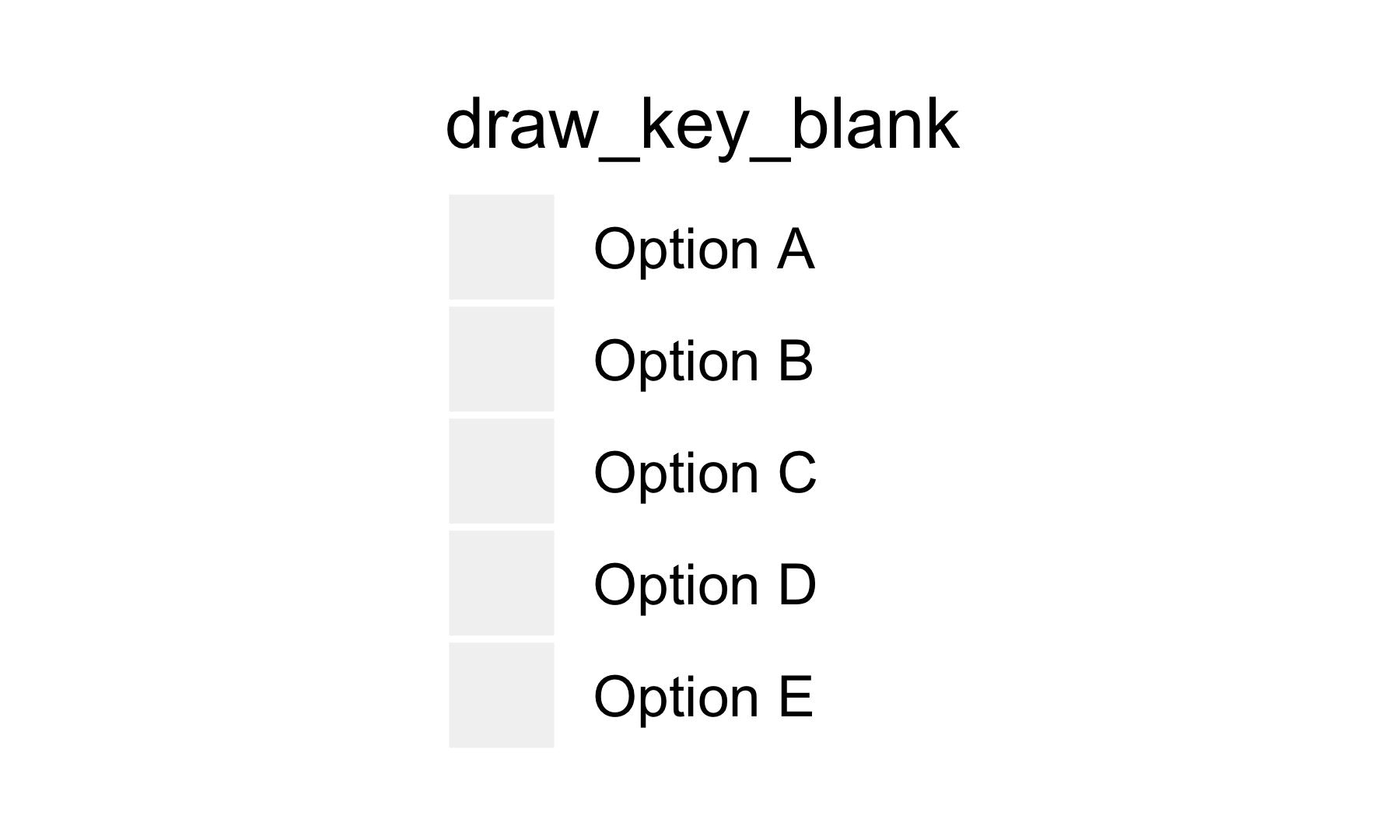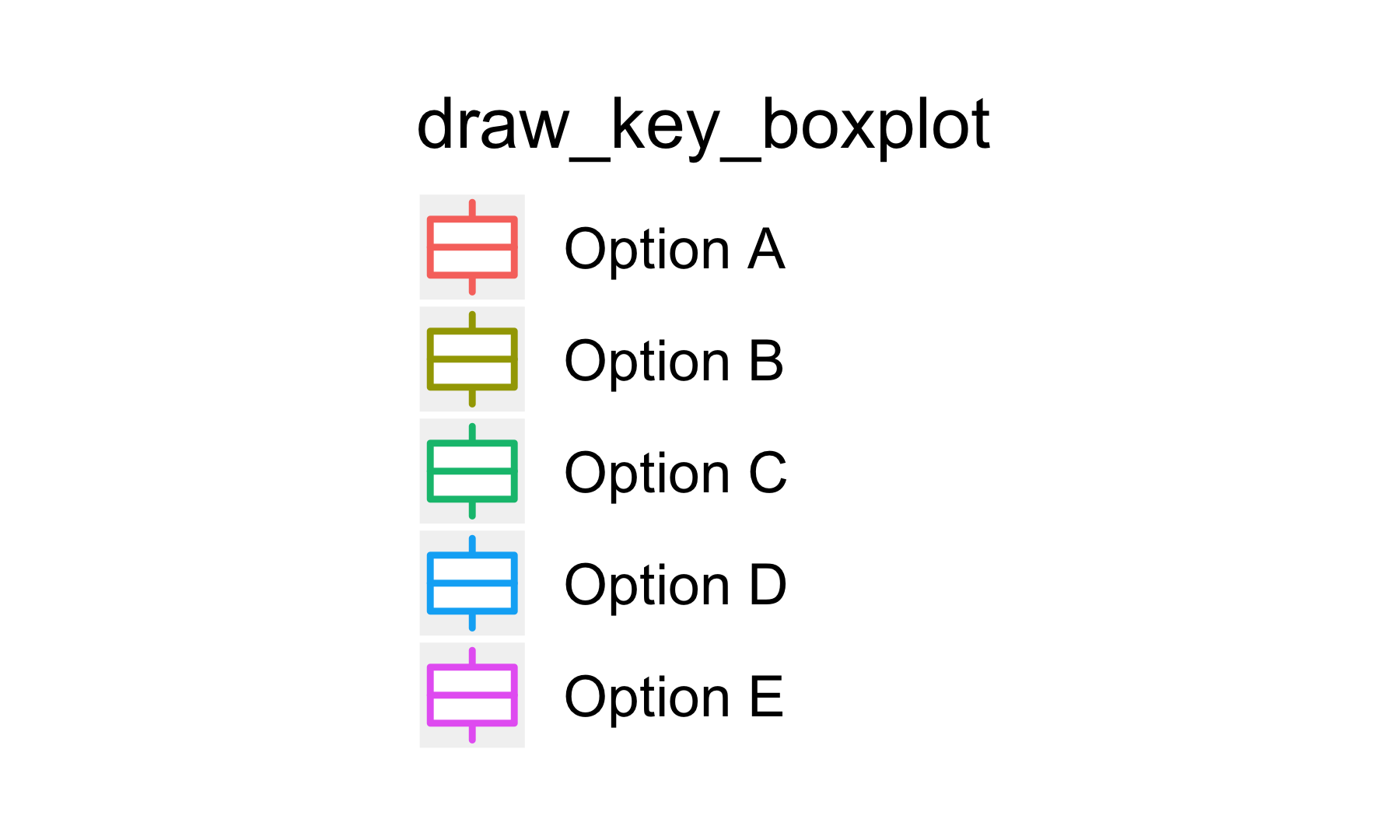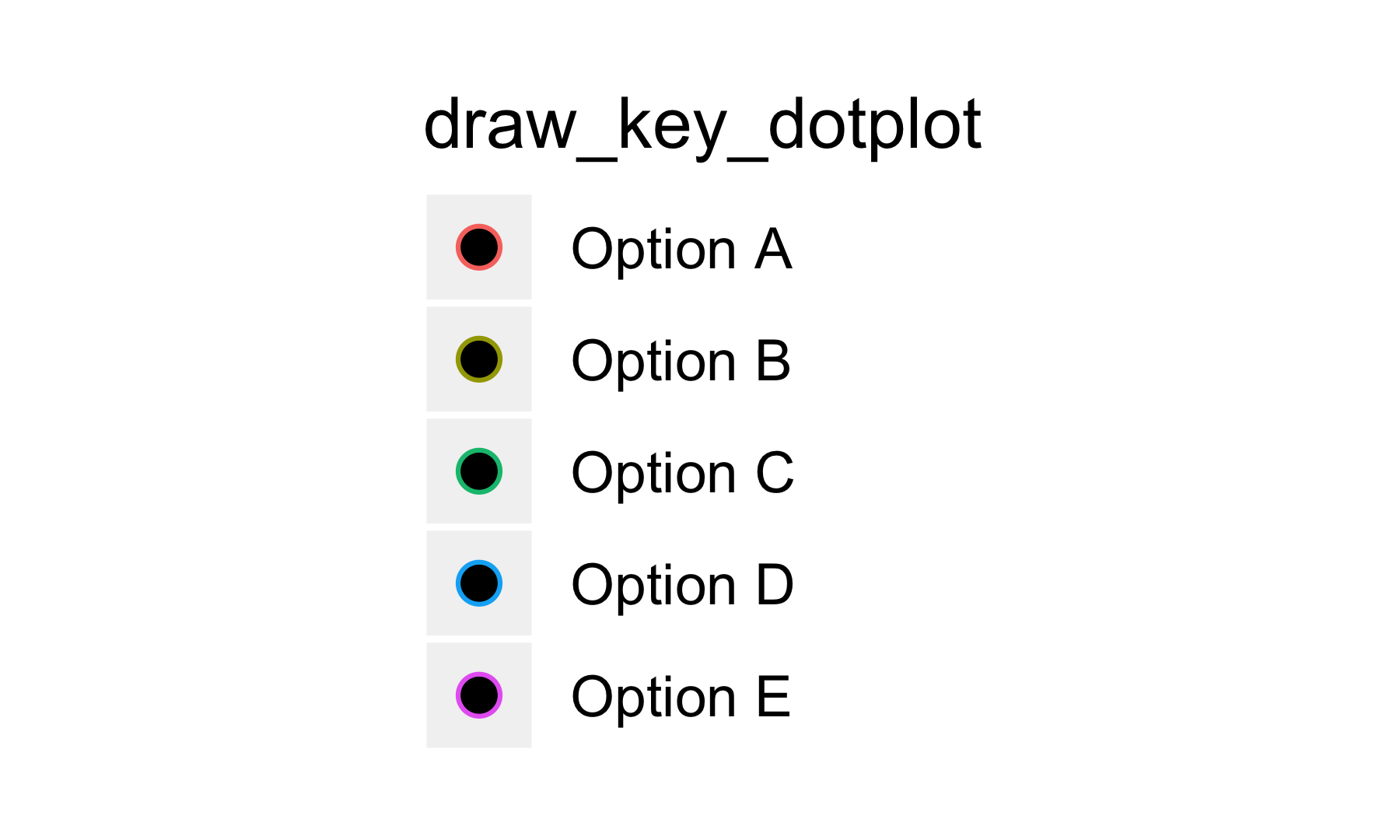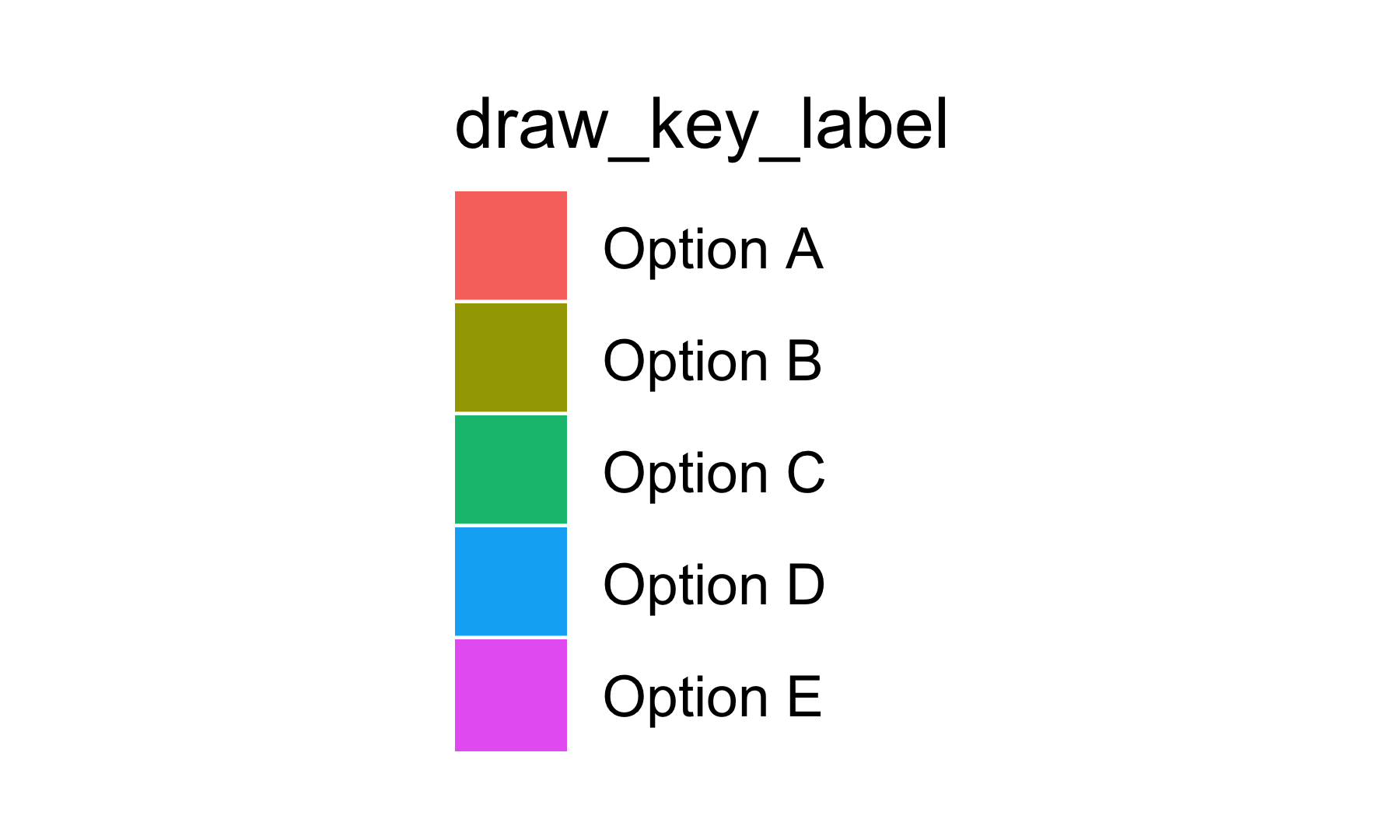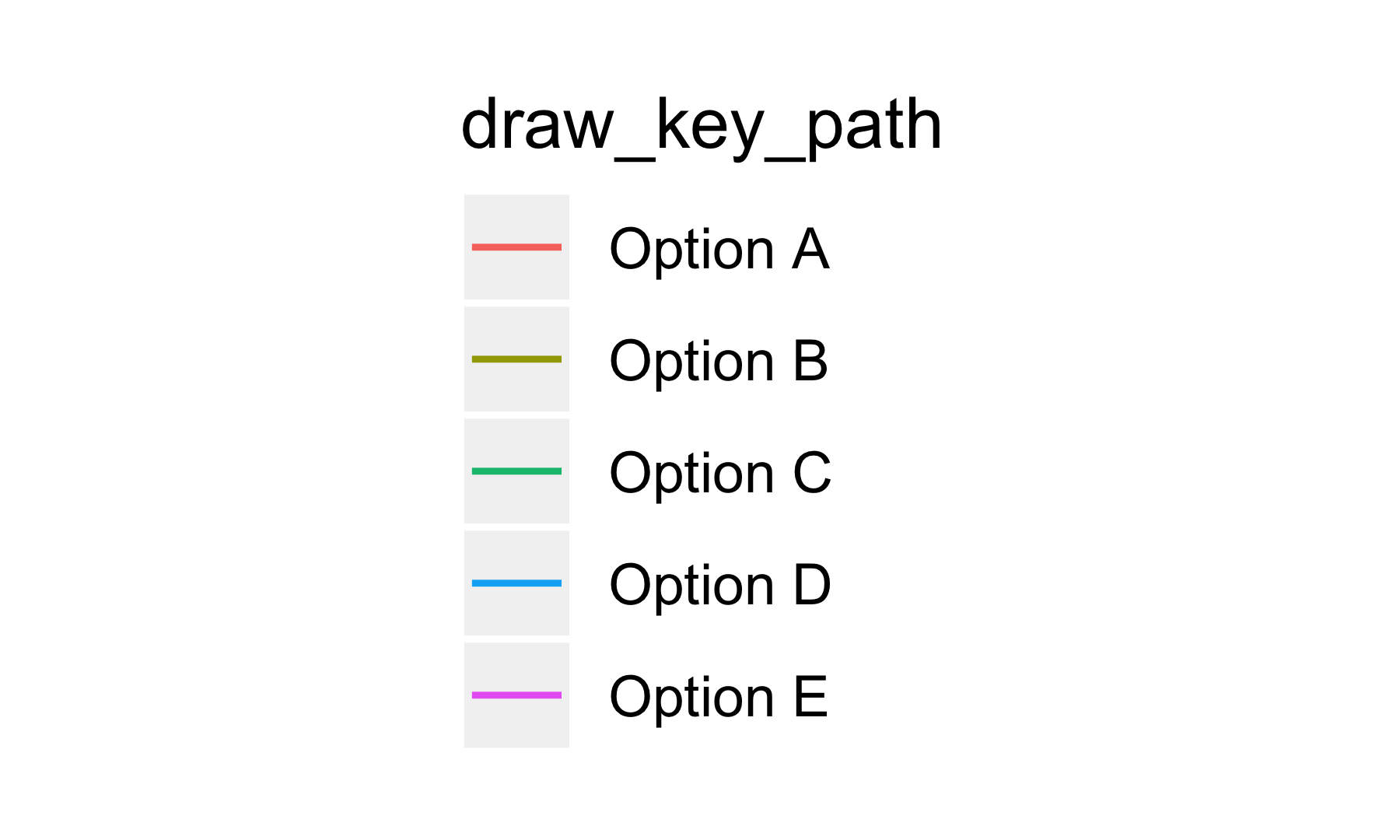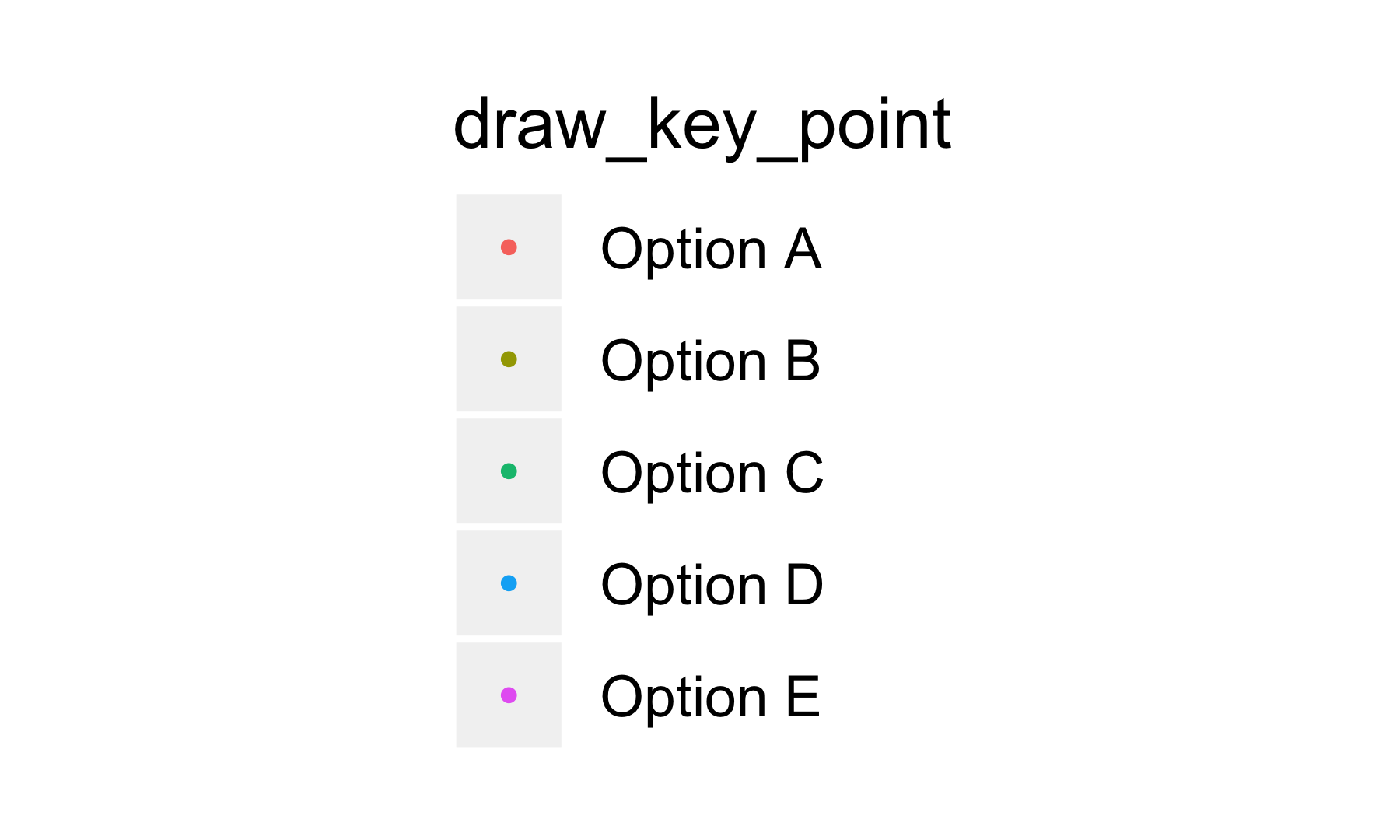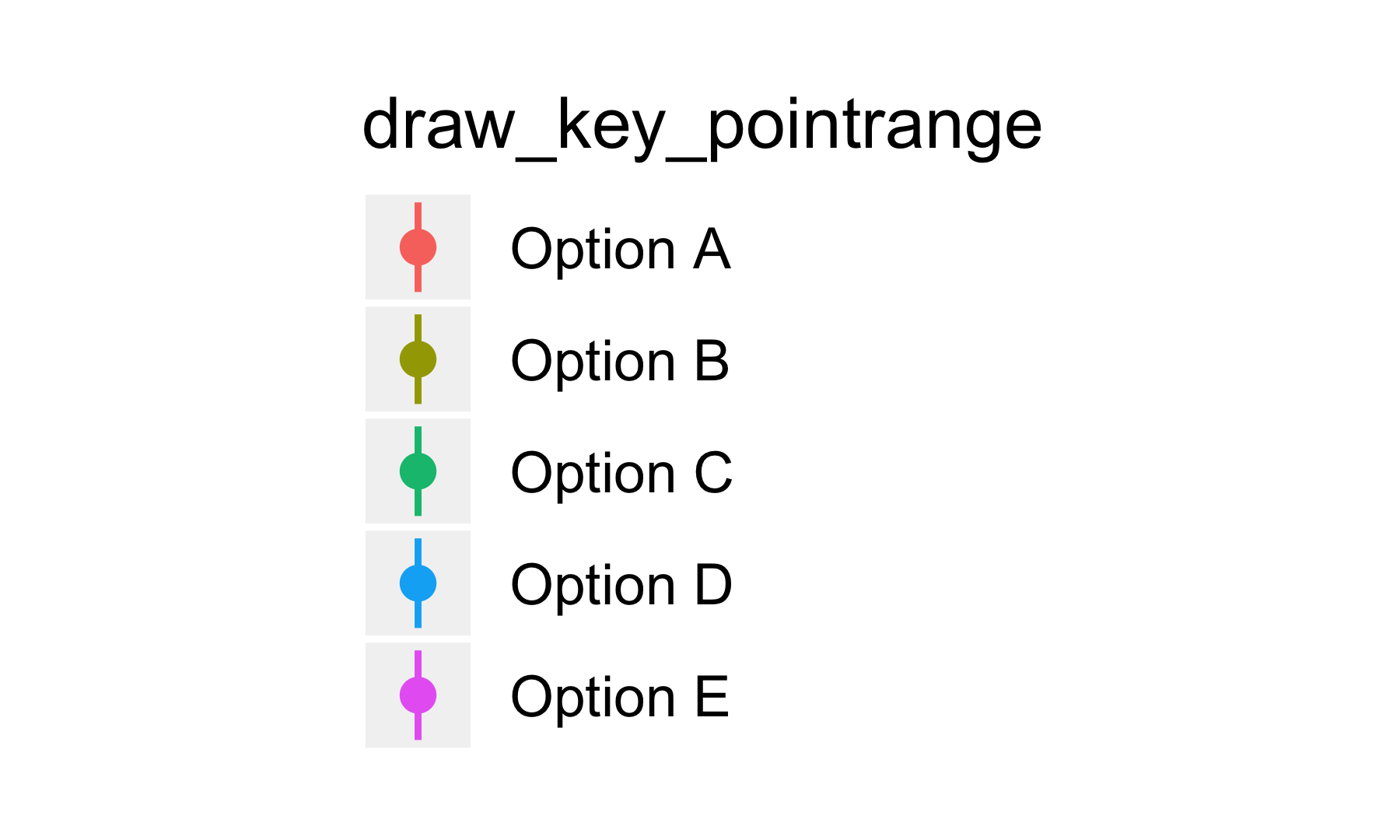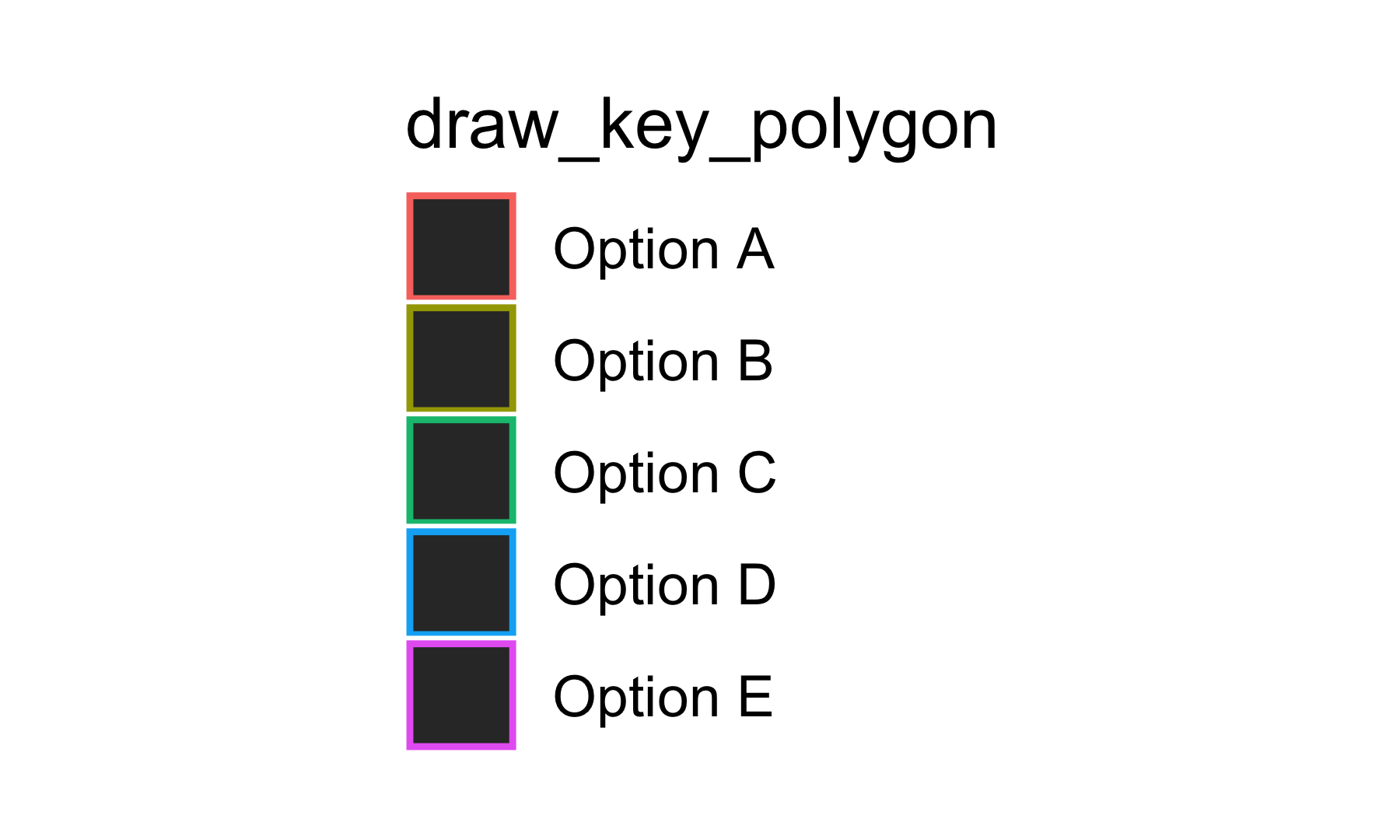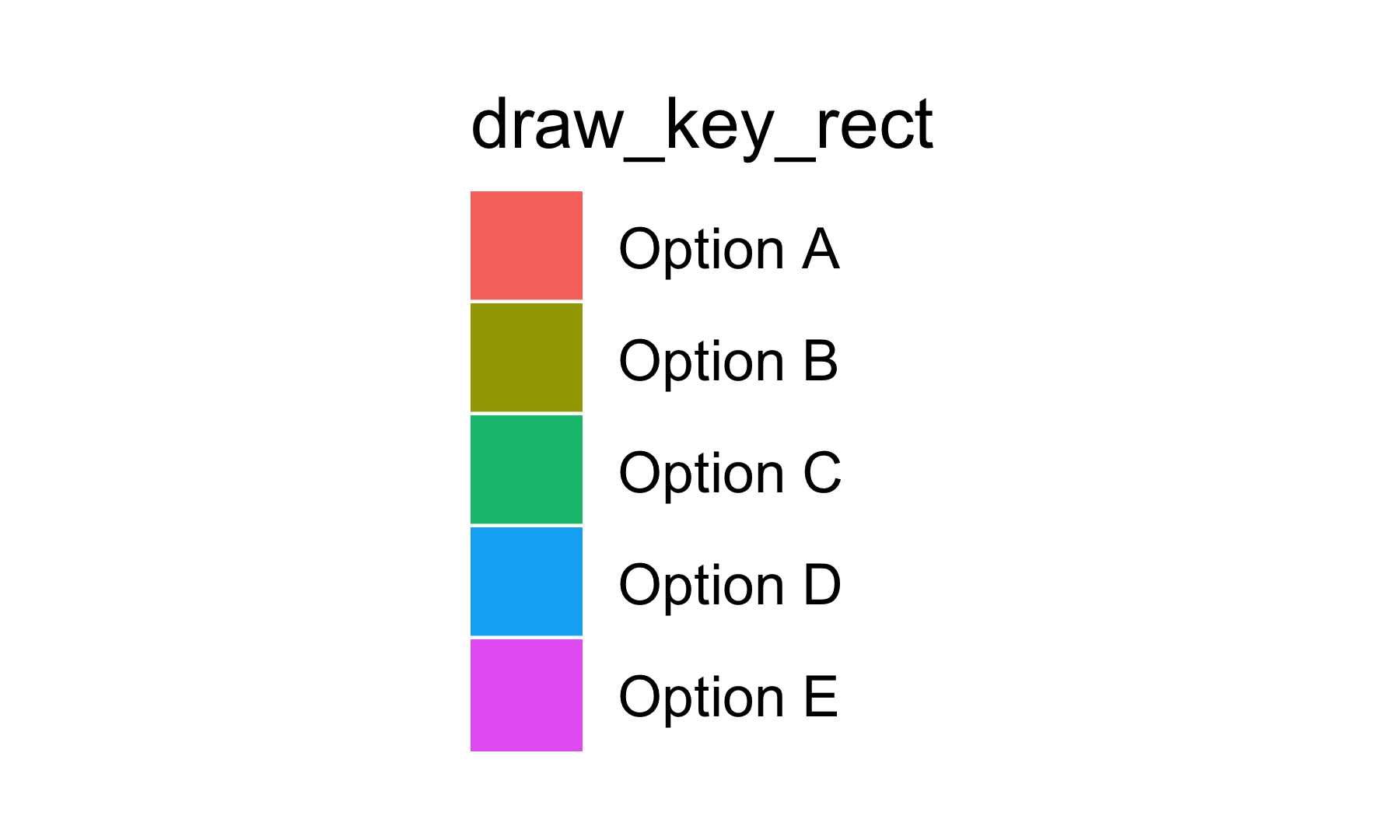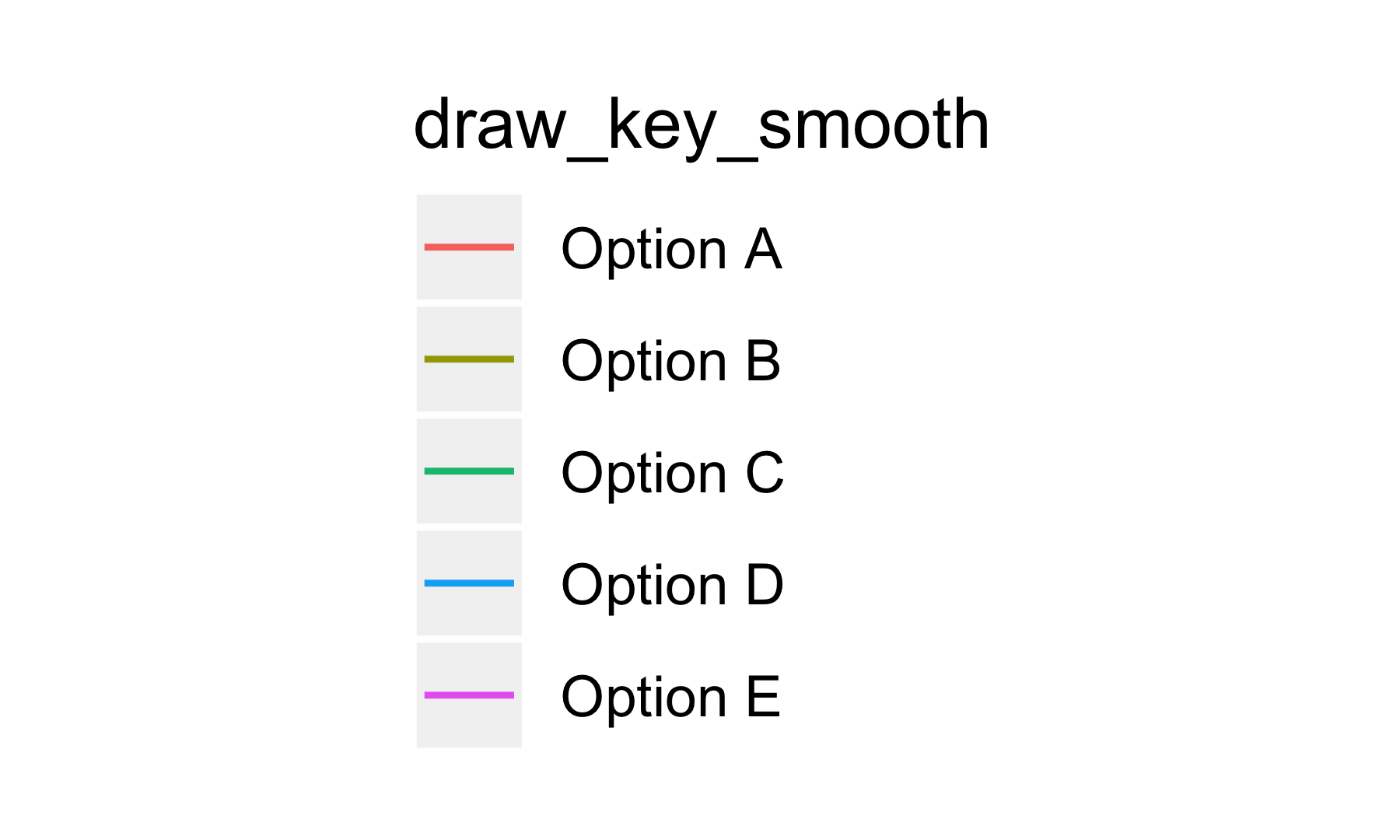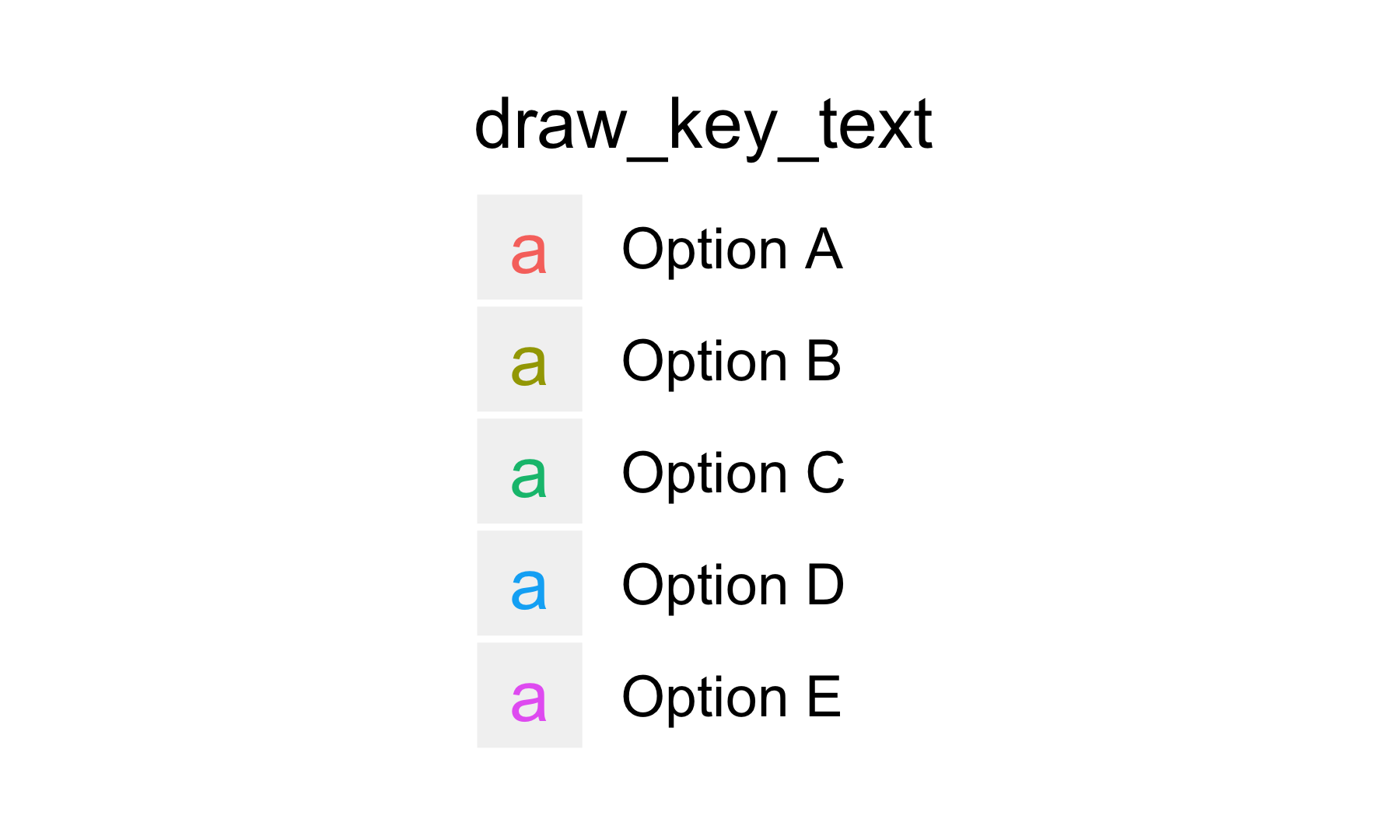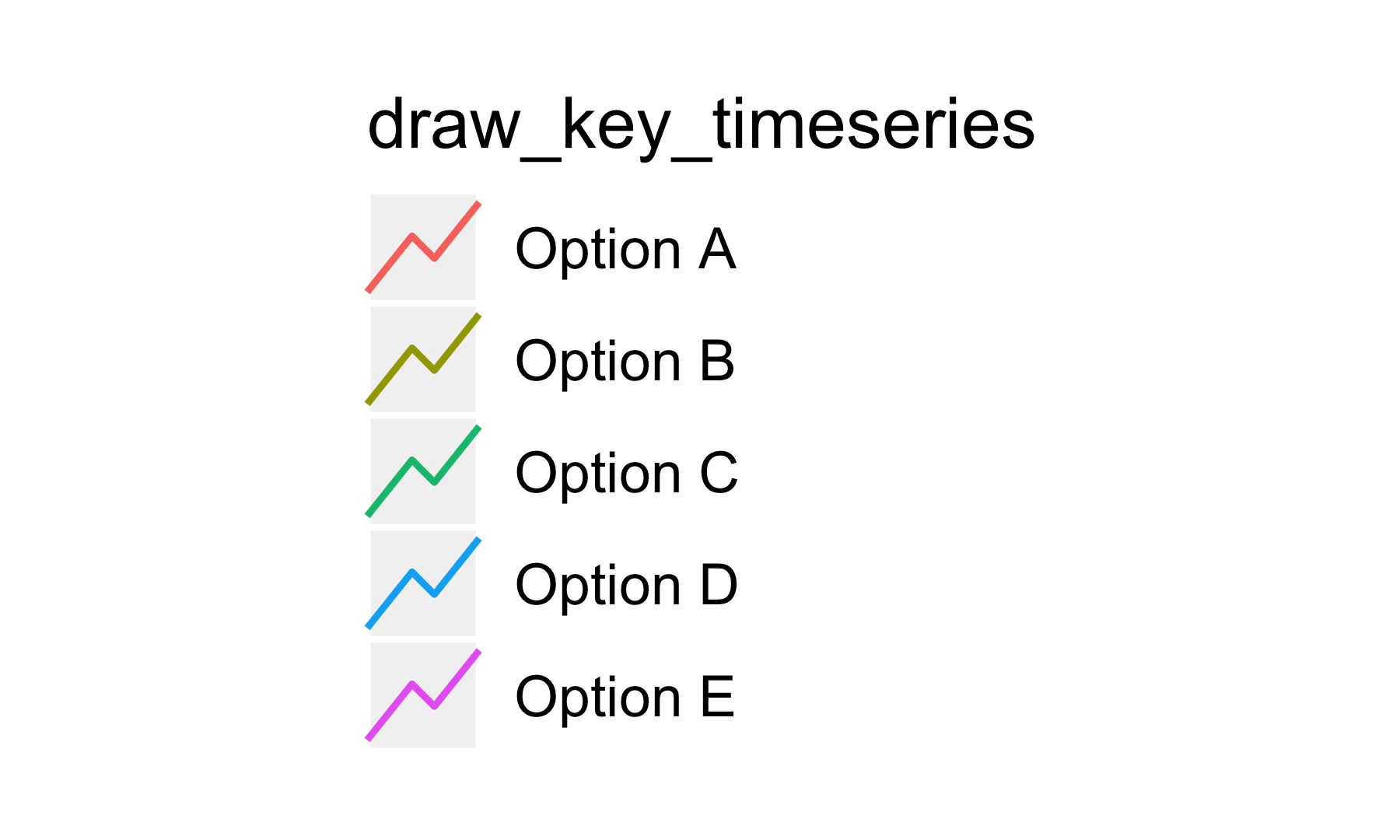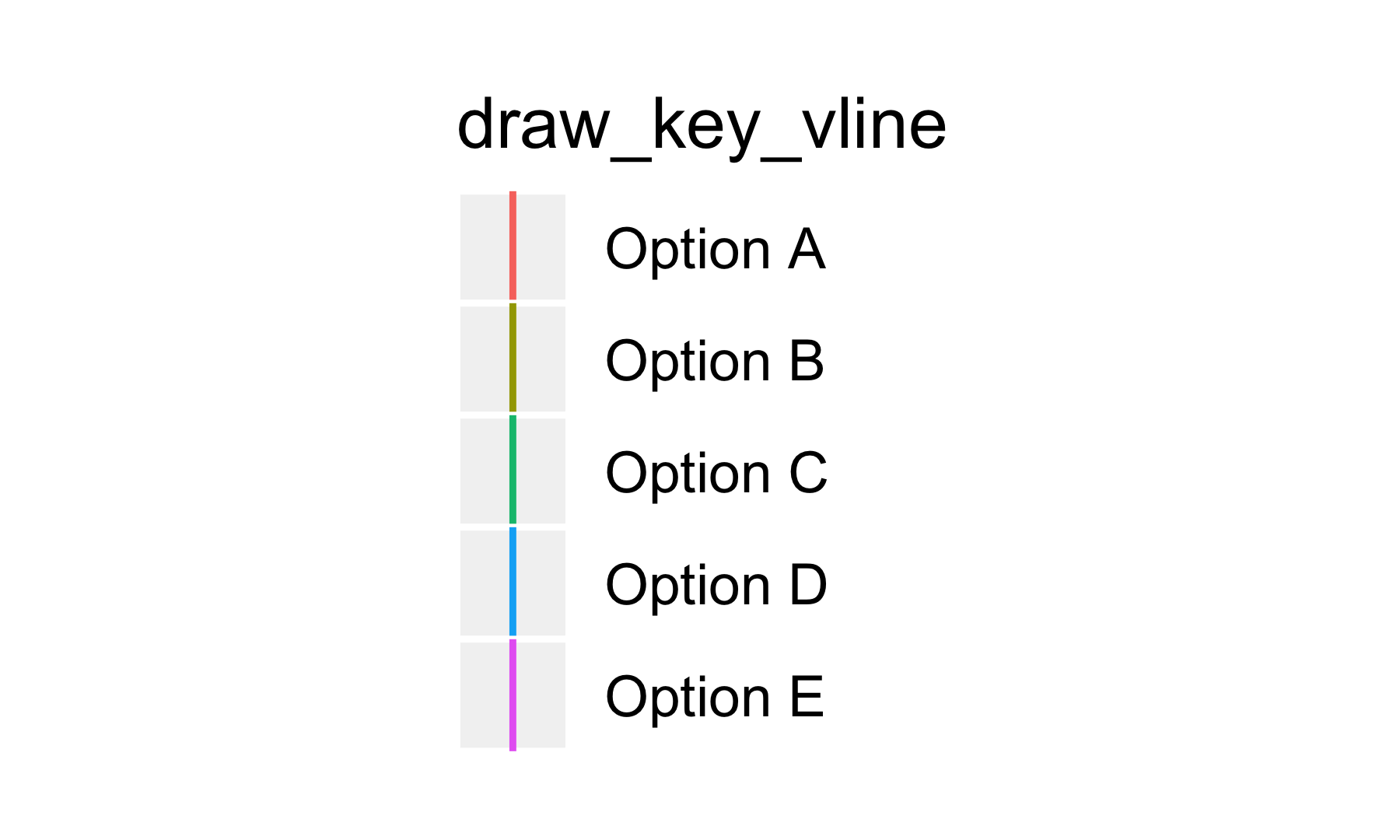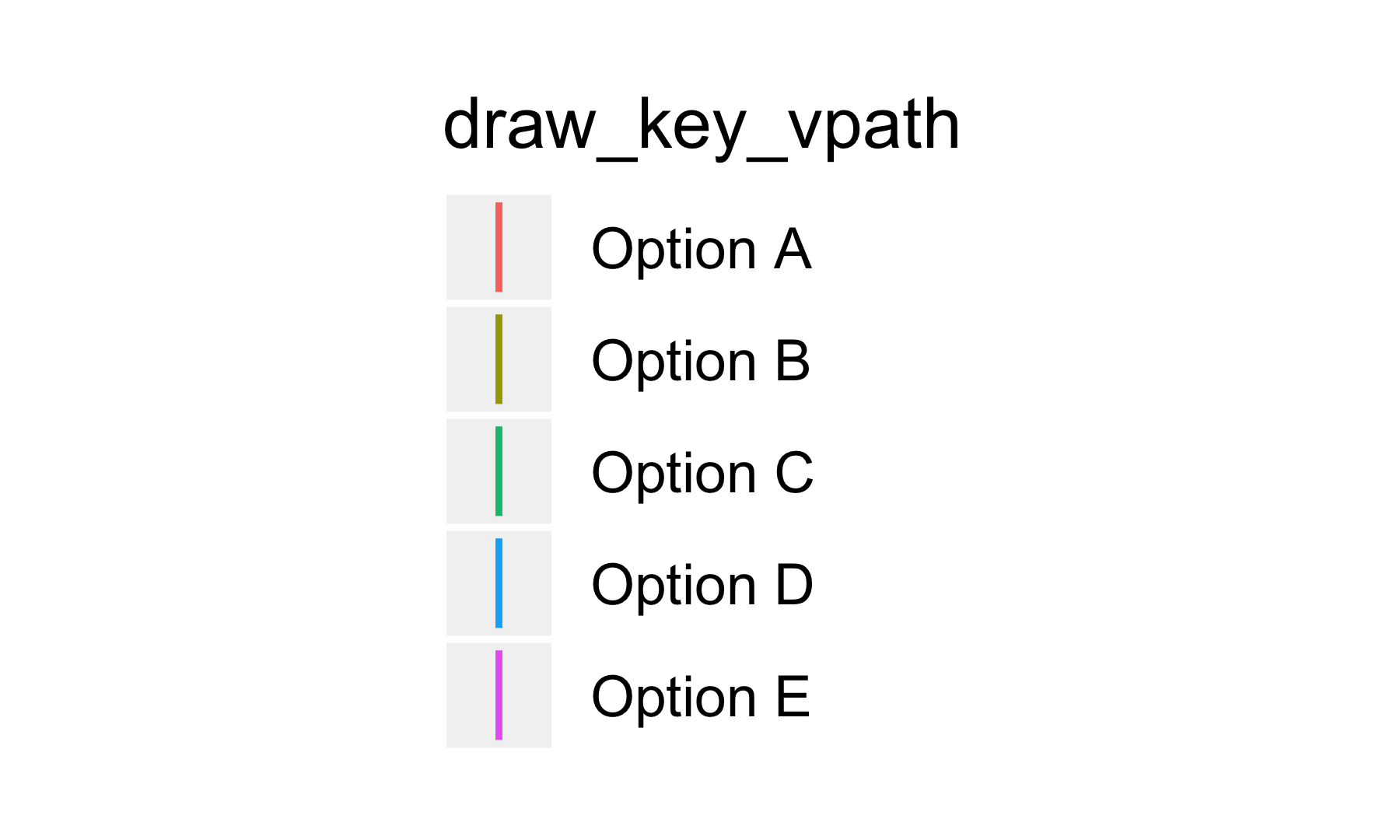# Custom glyph key

Since the `draw_key_*` function just return a grob, you can use spend some time and create your own custom glyphs! Taking inspiration from `draw_key_boxplot`

``````draw_key_boxplot
## function (data, params, size)
## {
##     grobTree(linesGrob(0.5, c(0.1, 0.25)), linesGrob(0.5, c(0.75,
##         0.9)), rectGrob(height = 0.5, width = 0.75), linesGrob(c(0.125,
##         0.875), 0.5), gp = gpar(col = data\$colour %||% "grey20",
##         fill = alpha(data\$fill %||% "white", data\$alpha), lwd = (data\$size %||%
##             0.5) * .pt, lty = data\$linetype %||% 1))
## }
## <bytecode: 0x7ffe31b7da80>
## <environment: namespace:ggplot2>``````

will I try to make a glyph by myself using both points and lines.

``````library(grid)
library(rlang)
draw_key_smile <- function(data, params, size) {
grobTree(
pointsGrob(0.25, 0.75, size = unit(.25, "npc"), pch = 16),
pointsGrob(0.75, 0.75, size = unit(.25, "npc"), pch = 16),
linesGrob(c(0.9, 0.87, 0.78, 0.65, 0.5, 0.35, 0.22, 0.13, 0.1),
c(0.5, 0.35, 0.22, 0.13, 0.1, 0.13, 0.22, 0.35, 0.5)),
gp = gpar(
col = data\$colour %||% "grey20",
fill = alpha(data\$fill %||% "white", data\$alpha),
lwd = (data\$size %||% 0.5) * .pt,
lty = data\$linetype %||% 1
)
)
}

ggplot(economics_long, aes(date, value01, colour = variable)) +
geom_line(key_glyph = draw_key_smile)``````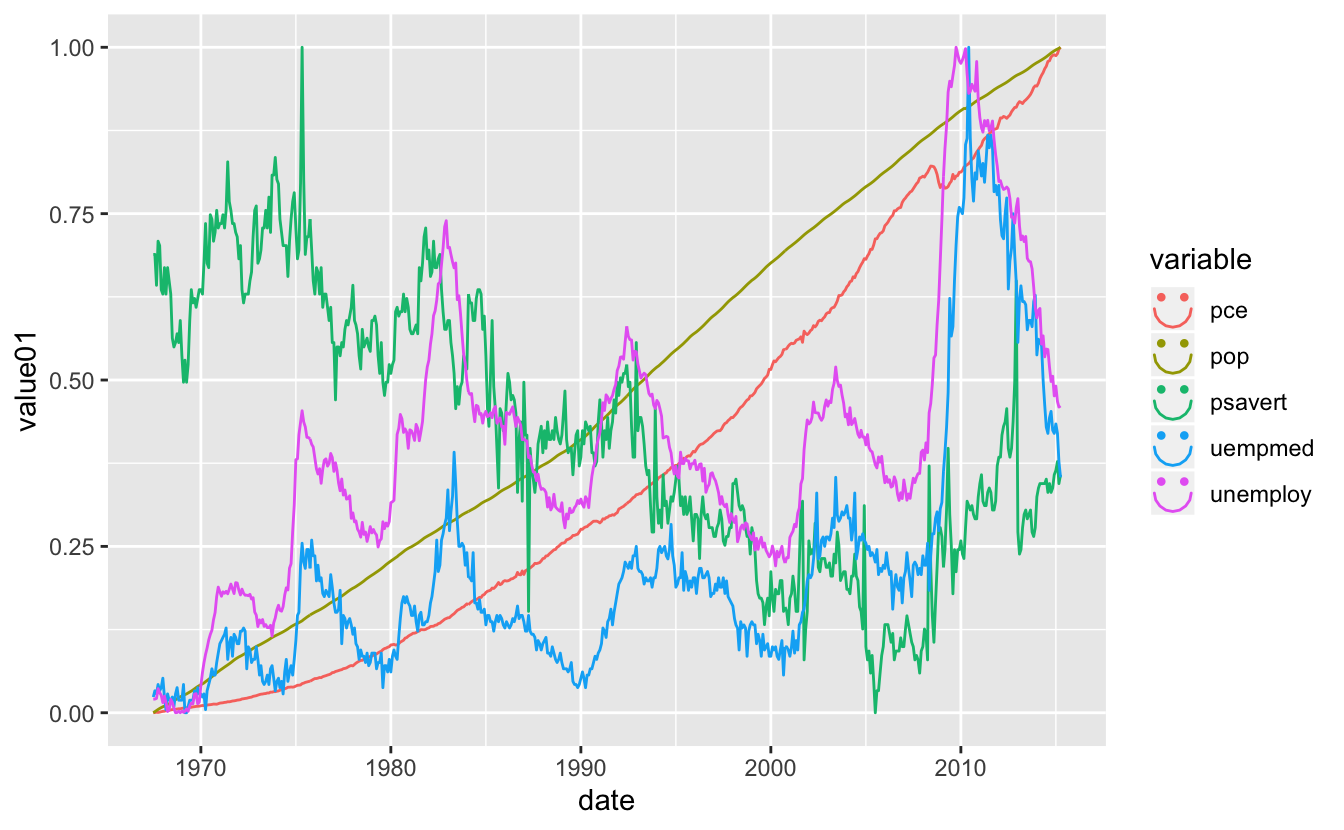And it looks so happy!

# Appendix

``````library(dplyr)
library(magrittr)
library(ggplot2)
library(grid)

draws <- ls(getNamespace("ggplot2"), pattern = "^draw_key_")

legend_fun <- function(x) {
ggg <- economics_long %>%
mutate(variable = factor(variable, labels = paste("Option", LETTERS[1:5]))) %>%
ggplot(aes(date, value01, colour = variable)) +
geom_line(key_glyph = get(x)) +
labs(color = x)

legend <- cowplot::get_legend(ggg)

grid.newpage()
grid.draw(legend)
}

purrr::walk(draws[1:12], legend_fun)
p <- ggplot(mtcars, aes(wt, mpg, label = rownames(mtcars))) +
geom_text(aes(colour = factor(ceiling(seq_len(nrow(mtcars)) %% 5), labels = paste("Option", LETTERS[1:5])))) +
labs(color = "draw_key_text")
legend <- cowplot::get_legend(p)

grid.newpage()
grid.draw(legend)
purrr::walk(draws[14:16], legend_fun)``````